De, X., Zhang, J., Yang, Y., Du, J., Guo, W., and Li, Z. (2021). "Fractal prediction of frictional force against the interior surface of forming channel coupled with temperature in a ring die pellet machine," BioResources 16(3), 4780-4797.

#### Abstract

For the biomass ring die pellet machine, the frictional force against the interior surface of the forming channel is the main cause for its frictional wear and also is key to the research of wear mechanism as well as its prediction. In this study, four ring die samples were used to measure and obtain data on their surface morphology. The fractal dimension D and fractal feature G were calculated using the Yardstick method, and lastly a fractal prediction model of sliding frictional force against the interior surface of forming channel was built, which was coupled with a fractal model of temperature distribution over friction surface. Numerical simulation, as well as friction-wear test were conducted to verify the accuracy of the model. The result showed that: when Ar < Arc, the slope of F was larger, which means the frictional force increased more rapidly, and the larger slope of FD represented a rapidly decreasing unit of frictional force. When true contact area Ar = 3.93%, Aa, FT, and FTD increased with the increase in temperature; FT increased rapidly at first and then gradually slowed down. When Ar was small, FTD increased sharply with the increase in temperature.

Fractal Prediction of Frictional Force against the Interior Surface of Forming Channel Coupled with Temperature in a Ring Die Pellet Machine

Xuehong De,a,# Jianchao Zhang,a,# Yang Yang,a Jianqiang Du,a,* Wenbin Guo,a and Zhen Li b

For the biomass ring die pellet machine, the frictional force against the interior surface of the forming channel is the main cause for its frictional wear and also is key to the research of wear mechanism as well as its prediction. In this study, four ring die samples were used to measure and obtain data on their surface morphology. The fractal dimension D and fractal feature G were calculated using the Yardstick method, and lastly a fractal prediction model of sliding frictional force against the interior surface of forming channel was built, which was coupled with a fractal model of temperature distribution over friction surface. Numerical simulation, as well as friction-wear test were conducted to verify the accuracy of the model. The result showed that: when Ar < Arc, the slope of F was larger, which means the frictional force increased more rapidly, and the larger slope of FD represented a rapidly decreasing unit of frictional force. When true contact area Ar = 3.93%, Aa, FT, and FTD increased with the increase in temperature; FT increased rapidly at first and then gradually slowed down. When Ar was small, FTD increased sharply with the increase in temperature.

Keywords: Biomass pelleting; Frictional force; Prediction; Fractal; Temperature coupling

Contact information: a: School of Mechanical and Electrical Engineering, Inner Mongolia Agricultural University, Hohhot, PR China; b: School of Mechanical Engineering, Inner Mongolia University of Science and Technology, Baotou, PR China; #: These authors contributed equally to this work;

* Corresponding author: djq968@163.com

INTRODUCTION

According to the IPCC (Intergovernmental Panel on Climate Change) assessment report, anthropogenic emissions, especially fossil fuel consumption, is the dominant cause for the rise in greenhouse gas concentration and global warming (Church et al. 2013). To keep the global temperature increase well below 2 °C or even 1.5 °C, if possible, as planned in the Paris Agreement, the UNEP (United Nations Environmental Program) requires most of the nations to reduce their emissions of greenhouse gases significantly. At the same time, the development of clean energy technology is urgently needed; biomass energy, for instance, is drawing global attention as a renewable, clean, and abundant energy source (Bamisile et al. 2020; Khan and Ulucak 2020; Kim et al. 2020; Sulaiman et al. 2020; Yan et al. 2020). The IEA (International Energy Agency), EIA (Energy Information Administration, USA), and many other research institutions researched the development of biomass resource technology to promote the global utilization of biomass energy (Gutierrez et al. 2020; Ong and Wu 2020; Schwerz et al. 2020).

Biomass energy utilization has achieved considerable gains in power generation and aviation fuel production, while the progress in the research of biomass pellet production has been relatively slow (Shahabuddin et al. 2020). During 2014 and 2017, the production of biomass pellet dropped 2.56% in the EU. China has been developing biomass pelleting equipment for decades. Among them, the ring-die pelleting machine has had fast development for a high production rate but still meets the problem of high energy consumption as well as high cost, resulting in limited marketing (Cong et al. 2013; Ning et al. 2016; Huo et al. 2020).

The fractal phenomenon is widespread in nature, such as mountains, coastlines, rough surface contours, etc. The fractal reveals the essence of a series of complex natural phenomena. It has played an important role in promoting the research of complex issues in many disciplines (Mandelbrot 1982; Zhang 1995; Paul 2000). In the theory of fractal, complex and chaos, objects have scale independency or self-similarity; thus fractal analysis is also an effective tool to quantitatively describe the friction and wear (Majumdar and Bhushan 1990; Ge 1997; Chen et al. 1998). In this study, the interior surface of the forming channel and the exterior surface of biomass pellet both have fractal characteristics, showing self-similarity.

The biomass ring-die pelleting machine can efficiently produce biomass pellets by compressing materials into the forming channels and squeezing them out at normal temperature, i.e. when no additional heating is applied to supplement frictional heating. During the compressing process, friction occurs between the biomass pellets and the interior surface of the forming channel. This friction causes wear of the interior surface and eventually leads to failure of the forming channel (Yan 2011; Jiang et al. 2013; Li et al. 2020). In this sense, the investigation of frictional force against the interior surface of the forming channel is fundamental to the analysis of contact interaction between the forming channel and the biomass pellet, while building an accurate fractal model of this sliding frictional force coupled with temperature is essential for the mechanism and prediction research of frictional wear of the forming channel in ring-die biomass pelleting machines (An et al. 2020; Liu et al. 2020; Shi et al. 2020; Xiao et al. 2020).

Because the microscopic rough surface of the forming channel in the ring die has fractal features, the contact model based on the surface fractal parameter can objectively represent the contact feature of a rough surface; consequently, the calculation formula of frictional force based on this is scientifically reasonable (Zhang et al. 2019; Huang et al. 2020; Watson et al. 2020). According to classical contact mechanics, when the solid body’s surface is subjected to both normal and tangential forces, and if the ratio of the two forces is smaller than 0.3, the yield occurs beneath the surface; and if the ratio is larger than 0.3, the yield occurs at the edge of a point of contact (Schweinhart 2020; Sun et al. 2020).

According to molecular mechanical friction theory, the interaction of the two friction surfaces under loads could be divided into mechanical interaction and molecular interaction (Zhang and Wen 2002; Ge and Zhu 2005; Xia et al. 2014). Molecular interaction produces resistance to sliding due to molecular adhesion resulting from molecular activity and molecular forces. The mechanical interaction also impedes the relative sliding movement due to mutual meshing, collision, elastic deformation, and the ploughing effect of micro-asperities. Therefore, the frictional force is the tangential component of combined forces of molecular interaction and mechanical interaction between the two contacting surfaces. The temperature increase of the contact surface due to friction heating has considerable influence on friction and wear characteristics. During the compression process of the ring-die pellet machine, the forming channel is subjected to increasing temperature, and the frictional force is changed accordingly (Zhang 2002; Cao 2015; Liu et al. 2016; Nieslony et al. 2018; Tan et al. 2020). Based on the kinematic analysis and the contact mechanics analysis of a contacting pair of micro asperities, this study, with consideration of both the influences of mechanical deformation and molecular interaction as well as the temperature field, derived a formula of frictional force using fractal theory. Furthermore, a fractal prediction model of sliding frictional force against the interior surface of the forming channel coupled with temperature in the ring die pellet machine was built based on the classic contact mechanics formula and M-B fractal model(Ge and Zhu 2005).

FRACTAL PREDICTION MODEL OF SLIDING FRICTIONAL FORCE AGAINST THE INTERIOR SURFACE OF FORMING CHANNEL

Analysis of Contact Condition of Frictional Surface

It was assumed that the forming channel and the biomass pellet are element O1 and O2 of the friction pair, respectively, and the corresponding contour lines of the contacting surface S1 and S2 before contact deformation happens are z1(x) and z2(x), respectively. As shown in Fig. 1, at the smallest scale, it is given that a pair of micro asperity i contacts at the point of B1 for a moment, and slides over a distance S within a length of time.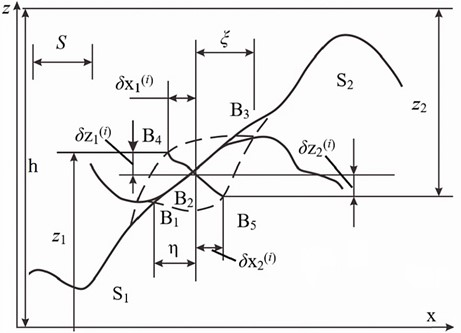Fig. 1. Contacting state of a pair of micro asperity after a relative sliding distance s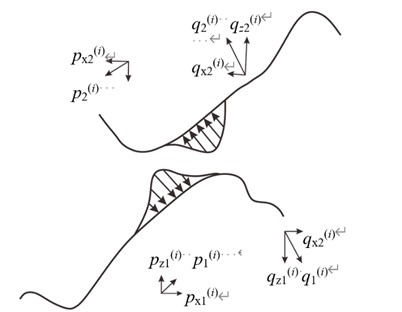Fig. 2. Force analysis of micro contacting surface

The dashed line shows the contour of shape without deformation, and Ai represents the contacting surface. The elastic deformation is δ1(i) and the plastic deformation is δ2(i). Their corresponding projections along the horizontal direction and vertical direction are δx1(i), δz1(i), and δx2(i), δz2(i), respectively. The change of vertical distance between two contact surfaces due to deformation or relative sliding is ∆h (Ge and Zhu 2005). See Eq. 1,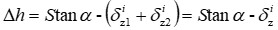(1)

where α is the inclination angle (°) of micro contact surface. Because the area of the micro contact surface of an individual micro asperity is small, an approximate value could be taken: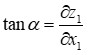(2)

For constant relative sliding speed Vx along the horizontal plane of the surface contact friction pair element, the relative sliding velocity Vz in the z direction depends on the the surface morphology and physical and mechanical properties. Given that Vx = ∂S / ∂t, Vz = ∂h / ∂t, taking partial derivative with respect to t for the Eq. 1 and substituting Eq. 2: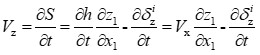(3)

Analysis of Micro Contact Mechanics

Because in the contact area, the micro asperity has both the mechanical deformation resistance q and the molecular adhesion resistance p, where the former one is perpendicular to the contact surface, while the latter one is parallel with it, then the overall contact pressure pz could be composed as:

pz(i)(x, y, z)=p(i)(x, y, z)+q(i)(x, y, z)

If the two contact surfaces can only move in the xz plane, regardless of the transitional movement and relative rotation along y-direction, and assuming that p and q are independent, then p and q can be decomposed in the x and z directions as,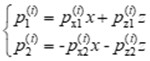(4)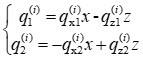(5)

where p1(i) and p2(i)q1(i) and q2(i) have the same value of projection with opposite signs in the corresponding direction. The relationship between slope and contact pressure at the points of contact is: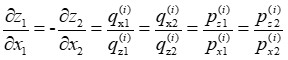(6)

Substituting Eq. 6 into Eq. 3, it becomes: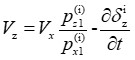(7)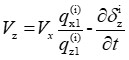(8)

Integrating both sides of Eq. 7, Eq. 8 on all the contact area in space Ai (i = 1, 2, …, n) and then seeking the summation, therefore: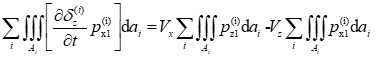(9)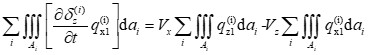(10)

Suppose that the z direction component and the x direction component of molecular interaction force on the whole contacting surface are Rz1 and Rz2, respectively. The z direction component and the x direction component of mechanical deformation resistance on the whole contacting surface are Tz1 and Tz2, respectively. Given that the left sides of Eqs. 9 and 10 are W(1) and W(2), respectively, then: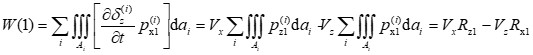(11)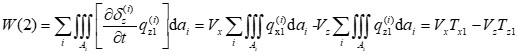(12)

Integrating both sides of Eq. 3 on all the contact area in space Ai (i = 1, 2, …, n) followed by the summation of it leads to the true contact area Ar of the two contacting surfaces,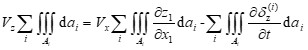(13)

which is,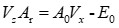(14)

where Ar is the true contact area of two contacting surfaces,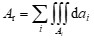; A0 is a constant,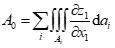; and E0 is the rate of change with respect to time of deformation along z direction,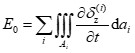.

Combining Eqs. 11, 12, and 14: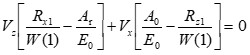(15)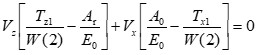(16)

Based on Eqs. 15 and 16, given that coefficient c = A0 / Ar, then 0 < c < 1, therefore: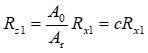(17)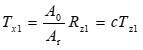(18)

Suppose that the normal load to the contacting surface of the forming channel and biomass pellet is FN, then FN = Rz1 + Tz1. Therefore, the frictional force F of biomass pellet against the interior surface of forming channel is as follows,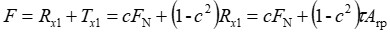(19)

where Arp is plastic contact area, Arp = Rx1 / τ (m2); and τ is the shear strength of forming channel material (Pa).

Because a rough surface has self-affine fractal features, according to the fractal representation of a rough surface and the theory of classical contact mechanics, a relation of plastic contact surface could be obtained as below (Chen et al. 2003),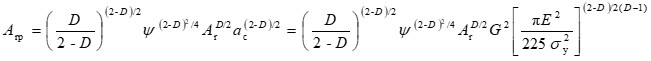(20)

where ac is the critical area at the moment of plastic deformation of point of contact (m2); Ψ is a coefficient that satisfies the transcendental equation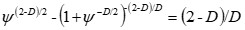; G is the coefficient of fractal dimensional feature, which reflects the surface profile amplitude of the forming channel in ring die; D is the fractal dimension of the surface profile; σy is the yield strength of the forming channel material in ring die; E is the composite elastic modulus,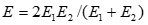(Pa); E1 is the elastic modulus of the forming channel in ring die (Pa); and E2 is the elastic modulus of biomass material (Pa).

Given that the unit bulging force exerted on the material by pellet is F0, therefore,

FN = F0Aa = F0lrd (21)

where lr is the sampling length of roughness measurement (m); d is the least contact width of friction material (m); and Aa is the theoretical contact area (m2).

Substituting Eqs. 20 and 21 into Eq. 19, then the fractal representation of sliding frictional force between the interior surface of the forming channel and pellet could be expressed as follows: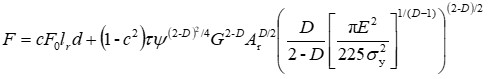(22)

According to the above, the frictional force on unit area, FD, is: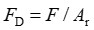(23)

Fractal Model of Temperature Distribution Over Friction Surface

During the compression process of the ring-die pellet machine, the forming channel is subjected to increasing temperature and the frictional force was also influenced accordingly. In this sense, it is necessary to have a coupled analysis of temperature and frictional force.

The velocity of the sliding movement of pellets in the forming channel is relatively slow (i.e., the instantaneous temperature change of the contact surface between the forming channel and the pellets is extremely small, so assuming the instantaneous contact temperature of the forming channel to be constant), and in the fractal area, temperature diffusion could be described by the temperature diffusion of static heat source on the semi-infinite body surface. The actual temperature Ts on the true contact area of the selected zone is (Wang and Komvopoulos 1994):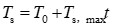(24)

The maximum temperature rise on the whole true contact surface, Ts,max, is: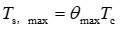(25)

The dimensionless maximum temperature rise in the fractal area, θmax, is: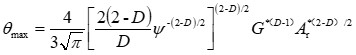(26)

The characteristic temperature rise Tc is: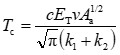(27)

Substituting Eqs. 25, 26, and 27 into Eq. 24 would be,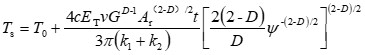(28)

where E is the composite elastic modulus with regard to temperature factor (Pa),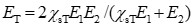; k1 is the thermal conductivity of the forming channel material in the ring die, W / (m × K); k2 is the thermal conductivity of biomass raw material, W / (m × K); v is the velocity of material sliding movement (m/s); T0 is the initial temperature of mould (°C); t is the time used for temperature rise from friction (s); and Ts is the actual temperature on the true contact area of the selected zone (°C).

According to the Chinese national standard GB 50017 (2017), the yield strength reduction coefficient of the forming channel material under high temperature, ηsT, is: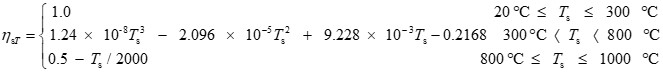(29)

According to the Chinese national standard GB 50017 (2017), the elastic modulus reduction coefficient of the forming channel material under high temperature, χsT, is: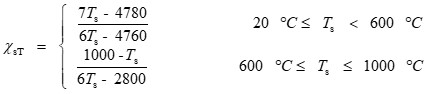(30)

Fractal Model of Frictional Force Coupled with Temperature

Combining Eqs. 23, 29, and 30, the fractal prediction model of frictional force FT coupled with temperature could be calculated as follows,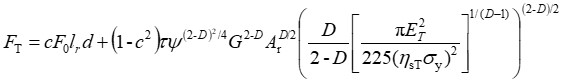(31)

where given that Ar = Mr1Aa, then the unit frictional force with regard to temperature, FTD, is: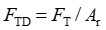(32)

FRACTAL PARAMETERS OF INTERIOR SURFACE PROFILE OF FORMING CHANNEL

The fractal theory could well explain the interior surface structure of the forming channel due to its apparent self-similarity and unsmooth characteristics (Ge and Zhu 2005). In this study, the Yardstick method was used to calculate the fractal dimension, and n yardsticks ri (i = 1, 2, …, n) were chosen to measure surface contour line. The length of the measured line for each yardstick was Li, so that a series of data was obtained (r1, L1), (r2, L2), …, (rn, Ln). Taking the linear regression of yardstick and contour line with the least square method in a log-log coordinate system, and the fractal dimension D could be calculated with the slope α of regression line:

D = 1 – α (33)

Then, the fractal feature parameter G could be obtained through the equation below,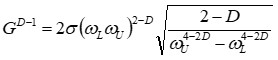(34)

where ωL is upper limit of cutoff low frequency of the surface profile, ωL = 1 / L; L is the sampling length of the surface profile (m); ωU is the upper limit of cutoff high frequency of the surface profile, ωU = 1 / 2δ; δ is the resolution of the profile, 0.001; σ is the standard deviation, 0.05.

PARAMETERS SETTING AND EXPERIMENTAL ANALYSIS

Experimental condition

The material of the tested forming channels were 45# steel (sample 1), 40 Cr steel (sample 2), 65 Mn spring steel (sample 3), and GCr15 steel (sample 4), as shown in Fig. 3. All the samples had the same diameter Φ18 mm, thickness 15 mm, and surface roughness Ra 3.2 μm. Sample 1 was treated with normalization while samples 2, 3, and 4 reached hardness HRC50~60 after heat treatment. The biomass material used in the experiment was blended sawdust of pine wood and poplar wood, with granularity 1 to 3 mm, moisture content 12%, and bulk density 0.26 g/cm3.

The main instruments used were: friction-wear test bench (self-made, Fig. 3), JB-8C roughness meter (YDYQ Precision Instruments Co., LTD., Guangzhou, China), DHS-10A moisture meter (Lichen-BX Instrument Technology CO., Ltd., Shanghai, China), electronic weightmeter (AR224N, OHAUS Co., LTD., New Jersey, USA), 100-mL measuring cylinder (1 mL accuracy), standard test sieve with 3-mm sieve pore as per GB/T6003.1 (1997), Vernier caliper, chronograph (0.01 s accuracy), tension and pressure sensor (Hunan Firstrate Co., LTD., Hunan, China, range: 0-5V, Resistance strain gauge, range: 0 to 500 kg, sensitivity: 1± 20% mV/V), and thermo-detector (testo 835 – H1, Testo Co., LTD., Schwarzwald, Germany, Infrared thermometer, range: -50 to 600 °C, sensitivity:0.1 °C).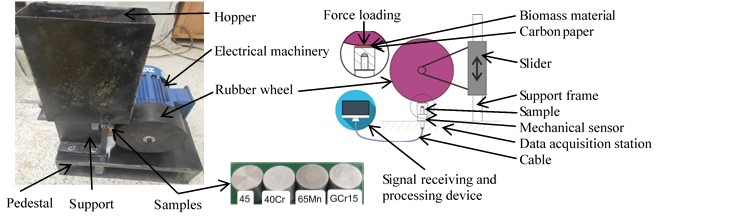Fig. 3. Friction wear test bench and measurement system of the pressure and the contact area

Methods and Materials

In this study, bulk density and moisture content of biomass material were measured. Roughness meter was used to measure and obtain data of the sample surface morphology of the forming channel, and then fractal dimension D was calculated using the yardstick method, followed by calculation of fractal feature G based on Eq. 34.

On the data acquisition platform, the relationship of real contact area Ar and contacting force is obtained between the sample and biomass materials. Ar is the area of carbon paper on the sample imprinted from rubber wheel pressure and contact force is read from mechanical sensor.

On the friction-wear test bench, a rubber roller rotated at 110 r/min driven by a 1.5 kW three-phase asynchronous motor and, together with falling sawdust, exerted pressure on the test samples so as to produce friction. The value of the pressure sensor was recorded every 5 min, and a total of 5 measurements were averaged to obtain the relationship between tested frictional force and true contact area Ar.

Bulk density of biomass material

Sieved material was put into a measuring cylinder through a funnel to the 100 mL line. The mass of the material was measured 5 times and averaged to calculate the bulk density.

Measurement of rough surface morphology

All the four samples of the forming channels were measured to obtain the data of the surface profile with roughness meter, and each for 5 times (Fig. 4 shows the surface morphology with Ra equal to 3.997 μm). The main parameters were: the arithmetical average deviation of the surface profile (Ra), the average spacing of irregularities (Rsm), the number of peaks (Rpc), the material portion of the profile (Mr1), the fractal dimension using the yardstick method (D), and the fractal feature parameter (G).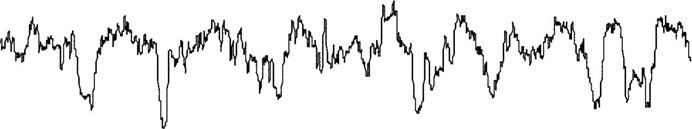Fig. 4. Rough morphology of the interior surface of the forming channel (Ra = 3.997 μm, 40 Cr )

RESULTS AND DISCUSSION

Table 1 shows the average value of surface morphology parameters as well as the calculated fractal dimension D using the yardstick method for each sample.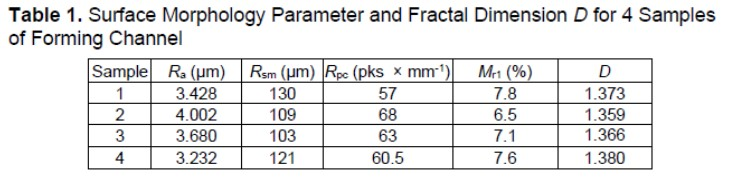Table 1 shows that when average roughness Ra was 3.428 μm for sample 1, the average value Mr1 was 7.8%, fractal dimension D average was 1.373, and the calculated fractal feature G was 1.55 × 10-6 m. For sample 2, Ra was 4.002 μm, the average value Mr1 was 6.5%, fractal dimension D average was 1.359, and the calculated fractal feature G was 0.82 × 10-6 m. For sample 3, Ra was 3.680 μm, the average value Mr1 was 7.1%, fractal dimension D average was 1.366, and the calculated fractal feature G was 1.13 × 10-6 m. While for sample 4, Ra was 3.232 μm, the average value Mr1 was 7.6%, fractal dimension D average was 1.380, and the calculated fractal feature G was 2.10 × 10-6 m.

PREDICTION AND ANALYSIS OF THE INTERIOR SURFACE OF FORMING CHANNEL

Prediction of Frictional Force

The initial parameters setting was as follows: F0 = 80 × 106 Pa, lr = 0.004 m, d = 1 × 10-6m, Ar = Mr1Aa, c = 0.2, E2 = 21.6 × 109 Pa, k2 = 0.15W / (m × °C), and v = 0.05 m / s. The fractal parameters settings of frictional force for each sample are shown in Table 2.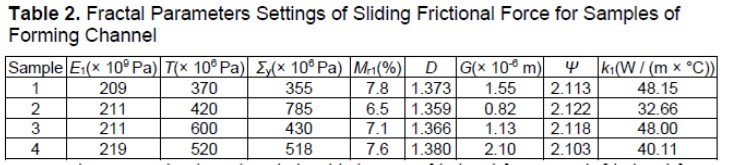Figures 5 and 6 show the relationship between frictional force F, unit frictional force FD, and true contact area Ar. It could be seen that, along with the increasing true contact area Ar , the frictional force F increased while the unit frictional force FD decreased. When Ar < Arc (critical true contact area), the slope of F was larger, which means the frictional force increased more rapidly, and the larger slope of FD, which indicated unit frictional force, decreased rapidly. When Ar > Arc, the slope of F decreased, it meant the increase of frictional force became slower, and the smaller slope of FD indicated a slower decrease of unit frictional force. This is consistent with the classical friction formula.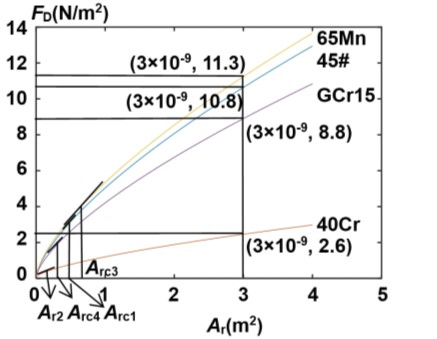Fig. 5. Relationship between frictional force F and true contact area Ar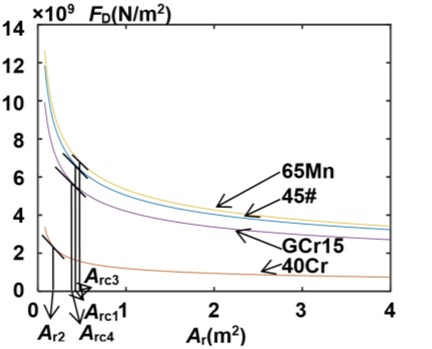Fig. 6. Relationship between unit frictional force FD and true contact area Ar

Figures 5 and 6 also show that the increment F of sample 3(65 Mn) was the largest, while that of sample 2 (40 Cr) was the least. Sample 2 had the largest reduction in FD, while that of sample 3 was the least. There was such a relation in terms of critical contact area Arc for the 4 samples: Arc2 (40 Cr) < Arc4 (GCr15) < Arc1 (45# steel) < Arc3 (65 Mn). This was due to mechanical characteristics of different materials. It also revealed that choosing 40 Cr steel as the forming channel material resulted in the least frictional force, suggesting that it would be more friction resistant and endurable.

Prediction of Frictional Force Coupled with Temperature

Figures 7 and 8 showed the relation curve between frictional force FT, unit frictional force FTD, and temperature T for sample 1 (45# steel) when true contact area Ar = 3.93% Aa. It could be seen that both FT and FTD increased with temperature increase. There were two inflection points: the slopes became precipitous at 635 °C, meaning the start of a rapid growth of FT and FTD while the slopes become smaller at 785 °C, meaning the growth slowed down thereafter. It was also indicated that when true contact area (Ar) is small, unit frictional force will be dramatically increased with temperature, thus resulting in severe friction and wear in the forming channel.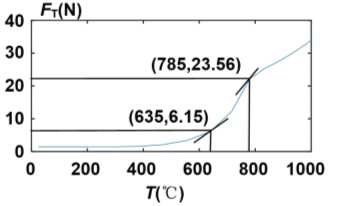Fig. 7. Relationship between frictional force FT and temperature (Ar = 3.93% Aa)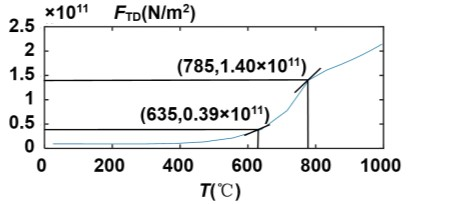Fig. 8. Relationship between unit frictional force FTD and temperature (45# steel, Ar = 3.93% Aa)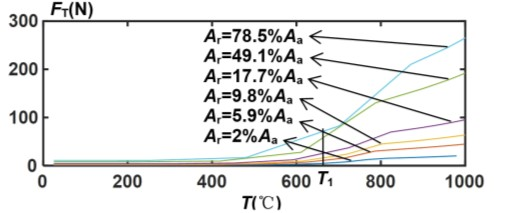Fig. 9. Relationship between frictional force FT and temperature (45# steel)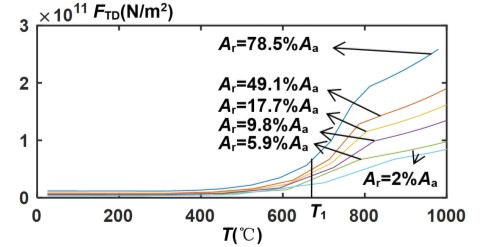Fig. 10. Relationship between unit frictional force FTD and temperature (45# steel)

Figures 9 and 10 show the relation curve between FT, FTD, and T for sample 1 (45# steel) with different true contact area Ar. It could be seen that FT and FTD increased with Ar, and their increment grew with rising temperature. This means larger contact area increases frictional force as well as unit frictional force so as to aggravate the wear. This also explains the reason for a short life span of forming channel despite its soft abrasives wear (Kong 2010).

Figures 11 and 12 show the relation curve between FT, FTD, and T for all four samples when true contact area Ar = 2% Aa. It could be seen that sample 3 (65 Mn) had the largest increment in FT and FTD, while that of sample 2 (40 Cr) was the least. That means that under the circumstance of the same contact area, the 40 Cr steel forming channel had the least unit frictional force and its wear was accordingly small so that it was the most endurable.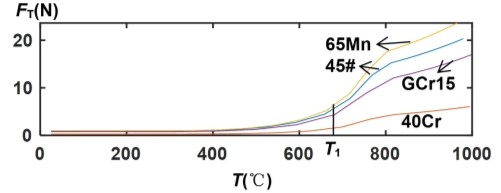Fig. 11. Relationship between frictional force FT and temperature(Ar=2%Aa)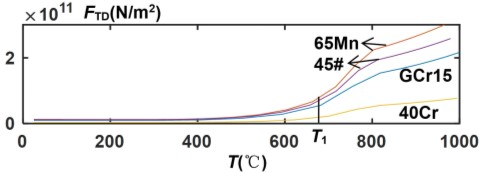Fig. 12. Relationship between unit frictional force FTD and temperature (Ar=2%Aa)

Test Verification of Sliding Frictional Force Coupled with Temperature

Table 3 shows the comparison of average unit sliding frictional force tested with friction-wear test bench and its prediction value under the circumstance of 200 °C and true contact area Ar = 2% Aa.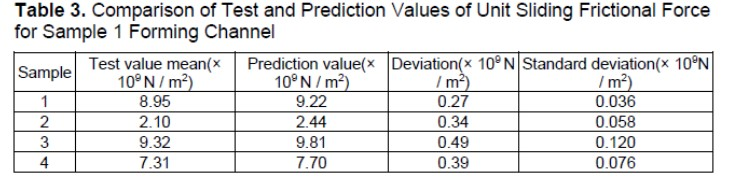It could be seen that the prediction values of FTD were slightly larger than the test values of the four samples. However, the overall difference and variation were small so that the coupled prediction was good. Hence, the fractal prediction model of sliding frictional force coupled with temperature can accurately predict the frictional force of forming channel.

CONCLUSIONS

In this study, four ring die samples (45# steel, 40 Cr steel, 65 Mn spring steel, and GCr15 steel) were used to measure and obtain data of their surface morphology with roughness meter. Then the fractal dimension D and fractal feature G were calculated using the yardstick method. Lastly, a fractal prediction model of sliding frictional force against the interior surface of forming channel was built based on classical contact mechanics and the M-B fractal contact model, which was coupled with the fractal model of temperature distribution over friction surface. Numerical simulation as well as friction – wear testing were conducted to verify the accuracy of the model. The main conclusions were as follows:

1. When the Ra was 3.428 μm for 45# steel, the fractal dimension D was 1.373 and the fractal feature G was 1.55 × 10-6 m. For 40 Cr steel, when Ra was 4.002 μm, D was 1.359, and G was 0.82 × 10-6 m. It was Ra 3.680 μm, D was 1.366, and G was 1.13 × 106 m for 65 Mn steel. For GCr15 steel, Ra was 3.232 μm, D was 1.380, and G was 2.10 × 10-6 m.
2. The prediction result of frictional force shows that along with the increasing true contact area Ar, the frictional force F increased while the unit frictional force FD decreased. When Ar < Arc, the slope of F was larger, which means the frictional force increased more rapidly, and the larger slope of FD represents a rapidly decreasing unit of frictional force. When Ar > Arc, the slope of F became smaller, which means the increase of frictional force became slower, and the smaller slope of FD represents a slower decrease of unit of frictional force.
3. The prediction result of frictional force coupled with temperature shows that when true contact area Ar = 3.93% Aa, FT and FTD increased with temperature increase; FT increased rapidly at first and gradually slowed down. When Ar was small, FTD increased sharply with temperature rise and the friction was intensified resulting in quick wear of the forming channel.
4. The prediction result was approximate to the test result so that an overall accurate prediction could be achieved by the model. Forming channel of 40 Cr steel had the least friction so that it is the most endurable of the 4 samples.

ACKNOWLEDGMENTS

The authors are grateful to Jianchao Zhang from Inner Mongolia Agricultural University, Hohhot, China, for help with laboratory analysis work. The authors also are grateful for the support of the National Natural Science Foundation of China (NSFC) Project (51766016; 51666016; 31960365) and the University Scientific Research Program of Inner Mongolia (NJZY20045).

REFERENCES CITED

An, Q., Suo, S. F., Lin, F. Y., Bai, Y. Z., Geng, H. X., and Shi, J. W. (2020). “A micro-contact model for rough surface of plane grinding,” Journal of Mechanical Engineering 56(7), 240-248. DOI: 10.3901/JME.2020.07.240

Bamisile, O., Huang, Q., Dagbasi, M., Adebayo, V., Adun, H., and Hu, W. H. (2020). “Steady-state and process modeling of a novel wind-biomass comprehensive energy system: An energy conservation, exergy and performance analysis,” Energy Conversion and Management 220, Article Number 113139. DOI: 10.1016/j.enconman.2020.113139

Cao Ya’nan. (2015). The Fractal Model and Evaluation on the Anisotropic and Rough Texture by Machining Operation, Master Thesis, Xi’an University of Technology, Xi’an, China.

Chen, G. A., Ge, S. R., and Wang, J. X. (1998). “Application of fractal geometry in tribology,” Tribology 18(2), 179-183. DOI: 10.3969/j.issn.0254-0150.2006.08.049

Chen, G. A., Zhu, Z. C., and Zhu, H. (2003). Research on the Wear of Process Equipment, Weapon Industry Press, Beijing, China

Church, J., Clark, P., Cazenave, A., Gregory, J., and Unnikrishnan, A. (2013). “Climate change 2013: The physical science basis. Contribution of working group I to the fifth assessment report of the Intergovernmental Panel on Climate Change,” (https://www.researchgate.net/publication/284695835_Climate_Change_2013_The_Physical_Science_Basis_Contribution_of_Working_Group_I_to_the_Fifth_Assessment_Report_of_the_Intergovernmental_Panel_on_Climate_Change) in: Intergovernmental Panel on Climate Change, New York, NY, USA, pp.total pages 121, Chapter 13 3-10.

Cong, H. B., Zhao, L. X., Yao, Z. L., Tian, Y. S., and Meng, H. B. (2013). “Analysis on capacity and energy consumption of biomass circular mould granulator,” Transactions of the Chinese Society for Agricultural Machinery 44(11), 144-149. DOI: 10.6041/j.issn.1000-1298.2013.11.026

Ge, S. R., and Zhu, H. (2005). Fractal of Tribology, 1st Ed., Machinery Industry Press, Beijing, China.

Ge, S. R. (1997). “The fractal behavior and fractal characterization of rough surfaces,” Tribology 17(1), 73-80. DOI: CNKI:SUN:MCXX.0.1997-01-010

GB50017. (2017). “Standard for design of steel structures,” General administration of quality supervision, inspection and quarantine of the People’s Republic of China, Beijing, China.

Gutierrez, A. S., Cabello Eras, J. J., Hens, L., and Vandecasteele, C. (2020). “The energy potential of agriculture agroindustrial, livestock, and slaughterhouse biomass wastes through direct combustion and anaerobic digestion. The case of Colombia,” Journal of Cleaner Production 269, Article Number 122317. DOI: 10.1016/j.jclepro.2020.122317

Huang, S. Z., Liu, J., and Shen, H. M. (2020). “Numerical study of the effect of initial roughness on tangential fretting behavior,” Journal of Sichuan University of Science & Engineering (Natural Science Edition) 33(3), 13-18. DOI: 10.11863/j.suse.2020.03.03

Huo, L. L., Zhao, L. X., Hao, Y. H., Meng, H. B., Yao, Z. L., Liu, Z., Liu, T. L., and Yuan, Y. W. (2020). “Quality standard system of densified biomass fuels at home and abroad,” Transactions of the Chinese Society of Agricultural Engineering 36(9), 245-254. DOI: 10.11975/j.issn.1002-6819.2020.09.028

Jiang, Q. H., Wu, K., Sun, Y., and Xia, X. F. (2013). “Wear mechanism analysis of ring die of pellet mill,” Transactions of the CSAE 29(22), 42-49. DOI: 10.3969/j.issn.1002-6819.2013.22.005

Khan, D., and Ulucak, R. (2020). “Linking biomass energy and CO2 emissions in China using dynamic autoregressive-distributed lag simulations,” Journal of Cleaner Production 250, Article Number 119533. DOI: 10.1016/j.jclepro.2019.119533

Kim, G. S., Choi, S. K., and Seok, J. H. (2020). “Does biomass energy consumption reduce total energy CO2 emission in the US?,” Journal of Policy Modeling 42(5), 953-967. DOI: 10.1016/j.jpolmod.2020.02.009

Kong, X. H. (2010). Experimental Study and Numerical Simulation of Ring Mold Wear of Biomass Solidification Molding, Doctoral Thesis, Northeast Forestry University, Harbin, China.

Li, Z., Wang, P., Liu, P., Gao, Y. H., and Wang, H. Q. (2020). “Fatigue life analysis of key components of ring molding machine based on fluid-solid coupling,” Journal of Forestry Engineering 5(1), 122-128. DOI:10.13360/j.issn.2096-1359.201811006

Liu, B.-B., Li, W.-F., Zhao, S.-Y., et al. (2016). “Research progress of metal surface feature extraction methods,” Electromechanical Technology 2016(2), 139-141. DOI: 10.3969/j.issn.1672-4801.2016.02.047

Liu, Y., Xia, T., Chen, Z. Y., and Yan, G. H. (2020). “Presentation and development of statistical contact model for rough surfaces,” Tribology 40(3), 395-406. DOI: 10.16078/j.tribology.2019191

Majumdar, A. A., and Bhushan, B. (1990). “Role of fractal geometry in roughness characterization and contact mechanics of surfaces,” ASME Journal of Tribology 112(2), 205-216. DOI: 10.1115/1.2920243

Mandelbrot, B. B. (1982). The Fractal Geometry of Nature, W. H. Freeman, New York, USA

Nieslony, P., Krolczyk, G. M., Wojciechowski, S., Chudy R., Zak, K., and Maruda, R. W. (2018). “Surface quality and topographic inspection of variable compliance part after precise turning,” Applied Surface Science 434, 91-101. DOI: 10.1016/j.apsusc.2017.10.158

Ning, T. Z., Ma, A. J., Yu, Y., and Chen, Z. J. (2016). “Problems and countermeasure analysis on biomass ring die pellet formation,” Journal of Chinese Agricultural Mechanization 37(1), 272-276. DOI: 10.13733/j.jcam.issn.2095-5553.2016.01.061

Ong, V. Z. Q., and Wu, T. Y. (2020). “An application of ultrasonication in lignocellulosic biomass valorisation into bio-energy and bio-based products,” Renewable and Sustainable Energy Reviews 132, Article Number 109924. DOI: 10.1016/j.rser.2020.109924

Paul, M. (2000). Fractal,Scaling and Growth Far from Equilibrium, Cambridge University Press, Cambridgeshire, UK, Tsinghua University Press, Beijing, China

Schweinhart, B. (2020). “Fractal dimension and the persistent homology of random geometric complexes,” Advances in Mathematics 372, 1-61. DOI: 10.1016/j.aim.2020.107291

Schwerz, F., Neto, D. D., Caron, B. O., Nardini, C., Sgarbosssa, J., Eloy, E., Behling, A., Elli, E. F, and Reichardt, K. (2020). “Biomass and potential energy yield of perennial woody energy crops under reduced planting spacing,” Renewable Energy 153, 1238-1250. DOI: 10.1016/j.renene.2020.02.074

Shahabuddin, M., Alam, T., Krishna, B. B., Bhaskar, T., and Perkins, G. (2020). “A review on the production of renewable aviation fuels from the gasification of biomass and residual wastes,” Bioresource Technology 312, Article Number 123596. DOI: 10.1016/j.biortech.2020.123596

Shi, X., Wang, W., Liu, K., Chen Y., Yang L., and Feng, S. Y. (2020). “Construction and contact analysis of micro-random rough surface contact finite element model,” Lubrication Engineering 45(5), 25-29. DOI: 10.3969/j.issn.0254-0150.2020.05.004

Sulaiman, C., Abdul-Rahim, A. S., and Ofozor, C. A. (2020). “Does wood biomass energy use reduce CO2 emissions in European Union member countries? Evidence from 27 members,” Journal of Cleaner Production 253, Article Number 119996. DOI: 10.1016/j.jclepro.2020.119996

Sun, H., Hou, M. X., Chen, C., and Ge, X. R. (2020). “Microstructure investigation of soft clay subjected to triaxial loading,” Engineering Geology 274, Article Number 105735. DOI: 10.1016/j.enggeo.2020.105735

Tan, W. B., Lan, G. S., and Zhang, X. L. (2020). “Modeling and simulation analysis of elliptic-plastic normal contact stiffness of joint surface based on anisotropic fractal theory,” Chinese Journal of Solid Mechanics (Accepted). DOI: 10.19636/j.cnki.cjsm42-1250/o3.2020.039

Wang, S., and Komvopoulos, K. A. (1994). “Fractal theory of the interfacial temperature distribution in the slow sliding regime: Part 1 – Elastic contact and heat transfer analysis,” ASME Journal of Tribology 116(4), 812-832. DOI: 10.1115/1.2927339

Watson, M., Lewis, R., and Slatter, T. (2020). “Improvements to the linear transform technique for generating randomly rough surfaces with symmetrical autocorrelation functions,” Tribology International 151, Article number 109487. DOI: 10.1016/j.triboint.2020.106487

Xia, X. F., Sun, Y., Wu, K., and Jiang Q. H. (2014). “Wear mechanism of ring die for straw briquetting machine,” Transactions of the CSAE 30(4), 32-38. DOI: 10.3969/j.issn.1002-6819.2014.04.005

Xiao, Y. Y., Wu, L. L., Luo, J., and Zhou, L. H. (2020). “Mechanical response of thin hard coatings under indentation considering rough surface and residual stress,” Diamond & Related Materials 108, Article Number 107991. DOI: 10.1016/j.diamond.2020.107991

Yan, P., Xiao, C. W., Xu, L., Yu, G. R., Li, A., Piao, S. L., and He, N. P. (2020). “Biomass energy in China’s terrestrial ecosystems: Insights into the nation’s sustainable energy supply,” Renewable and Sustainable Energy Reviews 127, Article Number 109857. DOI: 10.1016/j.rser.2020.109857

Yan, W. G. (2011). Research of Biomass Shaping with Open Mold in Natural Temperature, Doctoral Thesis, Beijing Forestry University, Beijing, China.

Zhang, Y. Q., Lu, H., and Zhang, X. B. (2019). “A normal contact stiffness model of machined joint surfaces considering elastic, elasto-plastic and plastic factors,” in: Proceedings of the Institution of Mechanical Engineers Part J: Journal of Engineering Tribology 234(7), 1-16. DOI: 10.1177/1350650119867801

Zhang, X. L., and Wen, S. H. (2002). “A fractal model of tangential contact stiffness of joint surfaces based on the contact fractal theory,” Journal of Agricultural Machinery 3, 91-97. DOI: 10.1007/s11766-996-0015-2

Zhang, X. L. (2002). Dynamic Characteristics of Machine Joint Surface and its Applications, 1st Ed., Press of Science and Technology of China, Beijing, China.

Zhang, J. Z. (1995). Fractal, Tsinghua University Press, Beijing, China

Article submitted: January 18, 2021; Peer review completed: April 3, 2021; Revised version received and accepted: April 30, 2021; Published: May 11, 2021.

DOI: 10.15376/biores.16.3.4780-4797